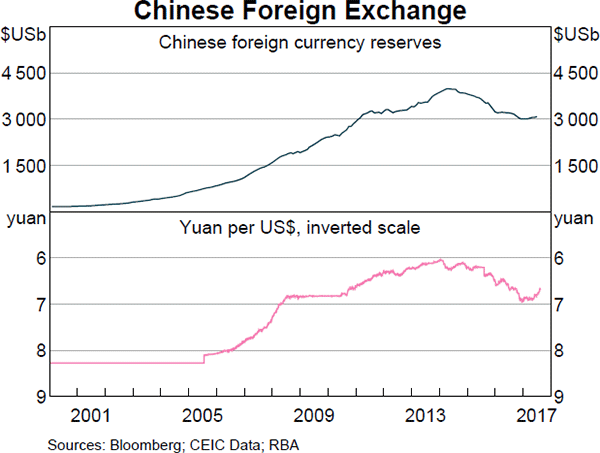# Fight Finance

#### CoursesTagsRandomAllRecentScores

A levered firm has a market value of assets of $10m. Its debt is all comprised of zero-coupon bonds which mature in one year and have a combined face value of$9.9m.

Investors are risk-neutral and therefore all debt and equity holders demand the same required return of 10% pa.

Therefore the current market capitalisation of debt $(D_0)$ is $9m and equity $(E_0)$ is$1m.

A new project presents itself which requires an investment of $2m and will provide a: •$6.6m cash flow with probability 0.5 in the good state of the world, and a
• -$4.4m (notice the negative sign) cash flow with probability 0.5 in the bad state of the world. The project can be funded using the company's excess cash, no debt or equity raisings are required. What would be the new market capitalisation of equity $(E_\text{0, with project})$ if shareholders vote to proceed with the project, and therefore should shareholders proceed with the project? What is the covariance of a variable X with itself? The cov(X, X) or $\sigma_{X,X}$ equals: A Chinese man wishes to convert AUD 1 million into Chinese Renminbi (RMB, also called the Yuan (CNY)). The exchange rate is 6.35 RMB per USD, and 0.72 USD per AUD. How much is the AUD 1 million worth in RMB? A stock has a beta of 1.5. The market's expected total return is 10% pa and the risk free rate is 5% pa, both given as effective annual rates. What do you think will be the stock's expected return over the next year, given as an effective annual rate? Alice, Bob, Chris and Delta are traders in the futures market. The following trades occur over a single day in a newly-opened equity index future that matures in one year which the exchange just made available. 1. Alice buys a future from Bob. 2. Chris buys a future from Delta. 3. Bob buys a future from Chris. These were the only trades made in this equity index future. What was the trading volume and what is the open interest? An equity index stands at 100 points and the one year equity futures price is 107. The equity index is expected to have a dividend yield of 3% pa. Assume that investors are risk-neutral so their total required return on the shares is the same as the risk free Treasury bond yield which is 10% pa. Both are given as discrete effective annual rates. Assuming that the equity index is fairly priced, an arbitrageur would recognise that the equity futures are: If the nominal gold price is expected to increase at the same rate as inflation which is 3% pa, which of the following statements is NOT correct? Observe the below graph of Chinese foreign exchange reserves held by the central bank, as well as the Chinese currency the Yuan (CNY, also called the Renminbi, RMB) against the US Dollar. Note the inverted y-axis scale on the Yuan exchange rate graph.Which of the below statements is NOT correct? A firm has 20 million shares, earnings (or net income) of$100 million per annum and a 60% debt-to-equity ratio where both the debt and asset values are market values rather than book values. Similar firms have a PE ratio of 12.

Which of the below statements is NOT correct based on a PE multiples valuation?

Find the Macaulay duration of a 2 year 5% pa semi-annual fixed coupon bond which has a \$100 face value and currently has a yield to maturity of 8% pa. The Macaulay duration is: